# ML | Data Preprocessing in Python

In order to derive knowledge and insights from data, the area of data science integrates statistical analysis, machine learning, and computer programming. It entails gathering, purifying, and converting unstructured data into a form that can be analysed and visualised. Data scientists process and analyse data using a number of methods and tools, such as statistical models, machine learning algorithms, and data visualisation software. Data science seeks to uncover patterns in data that can help with decision-making, process improvement, and the creation of new opportunities. Business, engineering, and the social sciences are all included in this interdisciplinary field.

## Data Preprocessing

Pre-processing refers to the transformations applied to our data before feeding it to the algorithm. Data preprocessing is a technique that is used to convert the raw data into a clean data set. In other words, whenever the data is gathered from different sources it is collected in raw format which is not feasible for the analysis.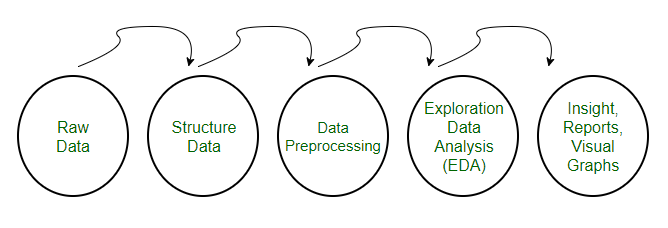Data Preprocessing

### Need of Data Preprocessing

• For achieving better results from the applied model in Machine Learning projects the format of the data has to be in a proper manner. Some specified Machine Learning model needs information in a specified format, for example, Random Forest algorithm does not support null values, therefore to execute random forest algorithm null values have to be managed from the original raw data set.
• Another aspect is that the data set should be formatted in such a way that more than one Machine Learning and Deep Learning algorithm are executed in one data set, and best out of them is chosen.

## Steps in Data Preprocessing

Step 1: Import the necessary libraries

## Python3

 `# importing libraries` `import` `pandas as pd` `import` `scipy` `import` `numpy as np` `from` `sklearn.preprocessing ``import` `MinMaxScaler` `import` `seaborn as sns` `import` `matplotlib.pyplot as plt`

## Python3

 `# Load the dataset` `df ``=` `pd.read_csv(``'Geeksforgeeks/Data/diabetes.csv'``)` `print``(df.head())`

Output:

```   Pregnancies  Glucose  BloodPressure  SkinThickness  Insulin   BMI
0            6      148             72             35        0  33.6  \
1            1       85             66             29        0  26.6
2            8      183             64              0        0  23.3
3            1       89             66             23       94  28.1
4            0      137             40             35      168  43.1

DiabetesPedigreeFunction  Age  Outcome
0                     0.627   50        1
1                     0.351   31        0
2                     0.672   32        1
3                     0.167   21        0
4                     2.288   33        1```

## Python3

 `df.info()`

Output:

```<class 'pandas.core.frame.DataFrame'>
RangeIndex: 768 entries, 0 to 767
Data columns (total 9 columns):
#   Column                    Non-Null Count  Dtype
---  ------                    --------------  -----
0   Pregnancies               768 non-null    int64
1   Glucose                   768 non-null    int64
2   BloodPressure             768 non-null    int64
3   SkinThickness             768 non-null    int64
4   Insulin                   768 non-null    int64
5   BMI                       768 non-null    float64
6   DiabetesPedigreeFunction  768 non-null    float64
7   Age                       768 non-null    int64
8   Outcome                   768 non-null    int64
dtypes: float64(2), int64(7)
memory usage: 54.1 KB```

As we can see from the above info that the our dataset has 9 columns and each columns has 768 values. There is no Null values in the dataset.

We can also check the null values using df.isnull()

## Python3

 `df.isnull().``sum``()`

Output:

```Pregnancies                 0
Glucose                     0
BloodPressure               0
SkinThickness               0
Insulin                     0
BMI                         0
DiabetesPedigreeFunction    0
Age                         0
Outcome                     0
dtype: int64```

#### Step 3: Statistical Analysis

In statistical analysis, first, we use the df.describe() which will give a descriptive overview of the dataset.

## Python3

 `df.describe()`

Output: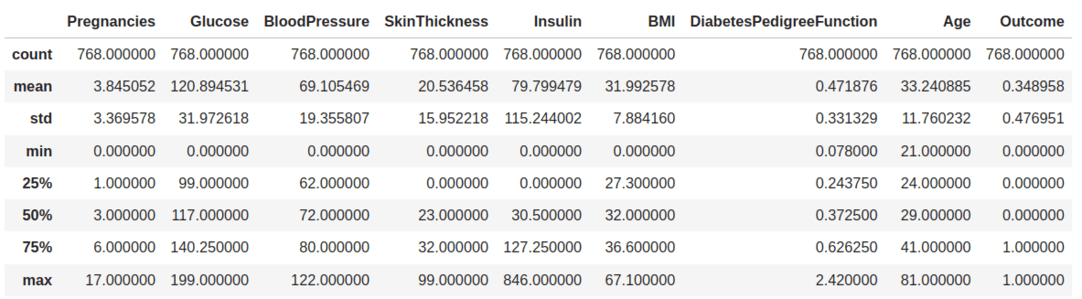Data summary

The above table shows the count, mean, standard deviation, min, 25%, 50%, 75%, and max values for each column. When we carefully observe the table we will find that. Insulin, Pregnancies, BMI, BloodPressure columns has outliers.

Let’s plot the boxplot for each column for easy understanding.

## Python3

 `# Box Plots` `fig, axs ``=` `plt.subplots(``9``,``1``,dpi``=``95``, figsize``=``(``7``,``17``))` `i ``=` `0` `for` `col ``in` `df.columns:` `    ``axs[i].boxplot(df[col], vert``=``False``)` `    ``axs[i].set_ylabel(col)` `    ``i``+``=``1` `plt.show()`

Output: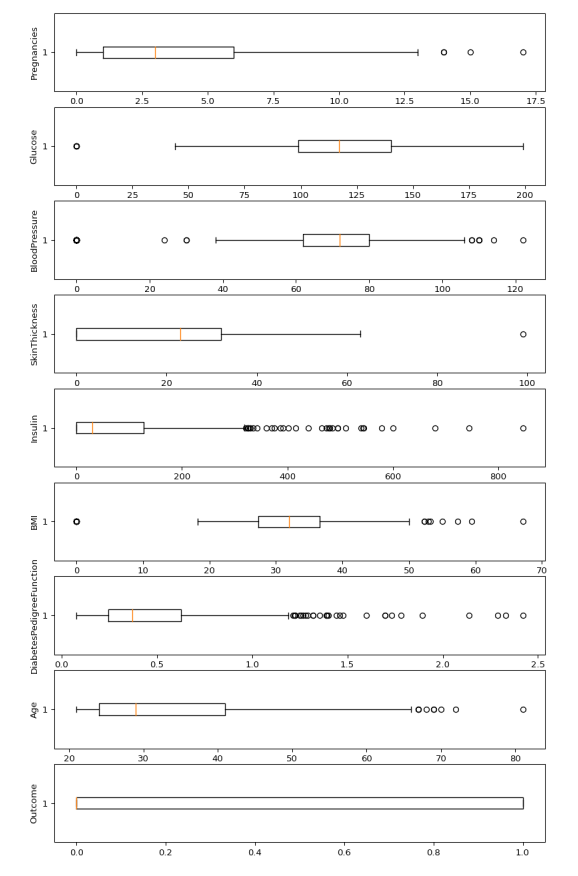Boxplots

from the above boxplot, we can clearly see that all most every column has some amounts of outliers.

## Python3

 `# Identify the quartiles` `q1, q3 ``=` `np.percentile(df[``'Insulin'``], [``25``, ``75``])` `# Calculate the interquartile range` `iqr ``=` `q3 ``-` `q1` `# Calculate the lower and upper bounds` `lower_bound ``=` `q1 ``-` `(``1.5` `*` `iqr)` `upper_bound ``=` `q3 ``+` `(``1.5` `*` `iqr)` `# Drop the outliers` `clean_data ``=` `df[(df[``'Insulin'``] >``=` `lower_bound) ` `                ``& (df[``'Insulin'``] <``=` `upper_bound)]`     `# Identify the quartiles` `q1, q3 ``=` `np.percentile(clean_data[``'Pregnancies'``], [``25``, ``75``])` `# Calculate the interquartile range` `iqr ``=` `q3 ``-` `q1` `# Calculate the lower and upper bounds` `lower_bound ``=` `q1 ``-` `(``1.5` `*` `iqr)` `upper_bound ``=` `q3 ``+` `(``1.5` `*` `iqr)` `# Drop the outliers` `clean_data ``=` `clean_data[(clean_data[``'Pregnancies'``] >``=` `lower_bound) ` `                        ``& (clean_data[``'Pregnancies'``] <``=` `upper_bound)]`     `# Identify the quartiles` `q1, q3 ``=` `np.percentile(clean_data[``'Age'``], [``25``, ``75``])` `# Calculate the interquartile range` `iqr ``=` `q3 ``-` `q1` `# Calculate the lower and upper bounds` `lower_bound ``=` `q1 ``-` `(``1.5` `*` `iqr)` `upper_bound ``=` `q3 ``+` `(``1.5` `*` `iqr)` `# Drop the outliers` `clean_data ``=` `clean_data[(clean_data[``'Age'``] >``=` `lower_bound) ` `                        ``& (clean_data[``'Age'``] <``=` `upper_bound)]`     `# Identify the quartiles` `q1, q3 ``=` `np.percentile(clean_data[``'Glucose'``], [``25``, ``75``])` `# Calculate the interquartile range` `iqr ``=` `q3 ``-` `q1` `# Calculate the lower and upper bounds` `lower_bound ``=` `q1 ``-` `(``1.5` `*` `iqr)` `upper_bound ``=` `q3 ``+` `(``1.5` `*` `iqr)` `# Drop the outliers` `clean_data ``=` `clean_data[(clean_data[``'Glucose'``] >``=` `lower_bound) ` `                        ``& (clean_data[``'Glucose'``] <``=` `upper_bound)]`     `# Identify the quartiles` `q1, q3 ``=` `np.percentile(clean_data[``'BloodPressure'``], [``25``, ``75``])` `# Calculate the interquartile range` `iqr ``=` `q3 ``-` `q1` `# Calculate the lower and upper bounds` `lower_bound ``=` `q1 ``-` `(``0.75` `*` `iqr)` `upper_bound ``=` `q3 ``+` `(``0.75` `*` `iqr)` `# Drop the outliers` `clean_data ``=` `clean_data[(clean_data[``'BloodPressure'``] >``=` `lower_bound) ` `                        ``& (clean_data[``'BloodPressure'``] <``=` `upper_bound)]`     `# Identify the quartiles` `q1, q3 ``=` `np.percentile(clean_data[``'BMI'``], [``25``, ``75``])` `# Calculate the interquartile range` `iqr ``=` `q3 ``-` `q1` `# Calculate the lower and upper bounds` `lower_bound ``=` `q1 ``-` `(``1.5` `*` `iqr)` `upper_bound ``=` `q3 ``+` `(``1.5` `*` `iqr)` `# Drop the outliers` `clean_data ``=` `clean_data[(clean_data[``'BMI'``] >``=` `lower_bound) ` `                        ``& (clean_data[``'BMI'``] <``=` `upper_bound)]`     `# Identify the quartiles` `q1, q3 ``=` `np.percentile(clean_data[``'DiabetesPedigreeFunction'``], [``25``, ``75``])` `# Calculate the interquartile range` `iqr ``=` `q3 ``-` `q1` `# Calculate the lower and upper bounds` `lower_bound ``=` `q1 ``-` `(``1.5` `*` `iqr)` `upper_bound ``=` `q3 ``+` `(``1.5` `*` `iqr)`   `# Drop the outliers` `clean_data ``=` `clean_data[(clean_data[``'DiabetesPedigreeFunction'``] >``=` `lower_bound) ` `                        ``& (clean_data[``'DiabetesPedigreeFunction'``] <``=` `upper_bound)]`

## Python3

 `#correlation` `corr ``=` `df.corr()`   `plt.figure(dpi``=``130``)` `sns.heatmap(df.corr(), annot``=``True``, fmt``=` `'.2f'``)` `plt.show()`

Output: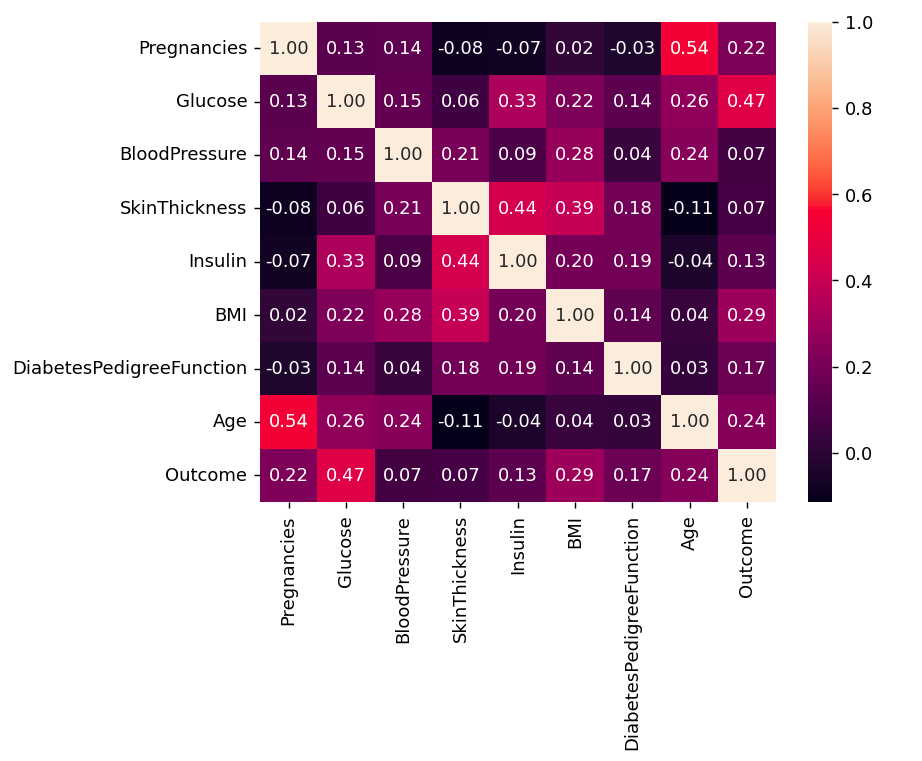Correlation

We can also camapare by single columns in descending order

## Python3

 `corr[``'Outcome'``].sort_values(ascending ``=` `False``)`

Output:

```Outcome                     1.000000
Glucose                     0.466581
BMI                         0.292695
Age                         0.238356
Pregnancies                 0.221898
DiabetesPedigreeFunction    0.173844
Insulin                     0.130548
SkinThickness               0.074752
BloodPressure               0.0```

## Python3

 `plt.pie(df.Outcome.value_counts(), ` `        ``labels``=` `[``'Diabetes'``, ``'Not Diabetes'``], ` `        ``autopct``=``'%.f'``, shadow``=``True``)` `plt.title(``'Outcome Proportionality'``)` `plt.show()`

Output: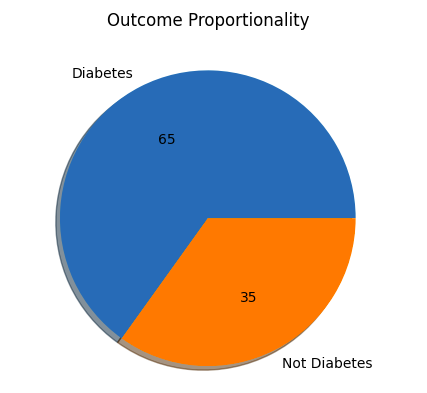Outcome Proportionality

## Python3

 `# separate array into input and output components` `X ``=` `df.drop(columns ``=``[``'Outcome'``])` `Y ``=` `df.Outcome`

#### Step 7: Normalization or Standardization

Normalization

• MinMaxScaler scales the data so that each feature is in the range [0, 1].
• It works well when the features have different scales and the algorithm being used is sensitive to the scale of the features, such as k-nearest neighbors or neural networks.
• Rescale your data using scikit-learn using the MinMaxScaler.

## Python3

 `# initialising the MinMaxScaler` `scaler ``=` `MinMaxScaler(feature_range``=``(``0``, ``1``))`   `# learning the statistical parameters for each of the data and transforming` `rescaledX ``=` `scaler.fit_transform(X)` `rescaledX[:``5``]`

Output:

```array([[0.353, 0.744, 0.59 , 0.354, 0.   , 0.501, 0.234, 0.483],
[0.059, 0.427, 0.541, 0.293, 0.   , 0.396, 0.117, 0.167],
[0.471, 0.92 , 0.525, 0.   , 0.   , 0.347, 0.254, 0.183],
[0.059, 0.447, 0.541, 0.232, 0.111, 0.419, 0.038, 0.   ],
[0.   , 0.688, 0.328, 0.354, 0.199, 0.642, 0.944, 0.2  ]])```

#### Standardization

• Standardization is a useful technique to transform attributes with a Gaussian distribution and differing means and standard deviations to a standard Gaussian distribution with a mean of 0 and a standard deviation of 1.
• We can standardize data using scikit-learn with the StandardScaler class.
• It works well when the features have a normal distribution or when the algorithm being used is not sensitive to the scale of the features

## Python3

 `from` `sklearn.preprocessing ``import` `StandardScaler`   `scaler ``=` `StandardScaler().fit(X)` `rescaledX ``=` `scaler.transform(X)` `rescaledX[:``5``]`

Output:

```array([[ 0.64 ,  0.848,  0.15 ,  0.907, -0.693,  0.204,  0.468,  1.426],
[-0.845, -1.123, -0.161,  0.531, -0.693, -0.684, -0.365, -0.191],
[ 1.234,  1.944, -0.264, -1.288, -0.693, -1.103,  0.604, -0.106],
[-0.845, -0.998, -0.161,  0.155,  0.123, -0.494, -0.921, -1.042],
[-1.142,  0.504, -1.505,  0.907,  0.766,  1.41 ,  5.485,```

Whether you're preparing for your first job interview or aiming to upskill in this ever-evolving tech landscape, GeeksforGeeks Courses are your key to success. We provide top-quality content at affordable prices, all geared towards accelerating your growth in a time-bound manner. Join the millions we've already empowered, and we're here to do the same for you. Don't miss out - check it out now!

Previous
Next